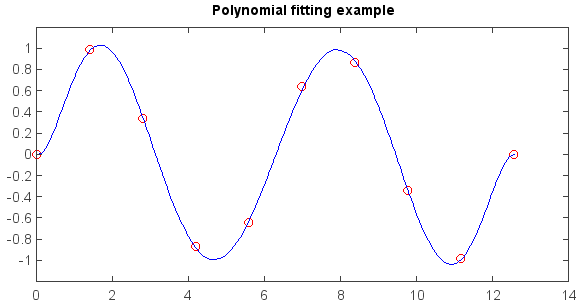# MeteoInfo 1.4.1 was released (2017-3-15)¶

A draft `series` module with a `Series` class was added in `numeric` package.

A `fitting` package was added in `numeric` package with functions of polynomail, power and exponent curve fitting.

Raster layer with none longlat projection in MeteoInfo can be saved to dist with `.prj` projection file. So it could be loaded correctly with project file.

```from mipylib.numeric import fitting

x = linspace(0, 4*pi, 10)
y = sin(x)

#Plot data points
plot(x, y, 'ro', fill=False, size=1)

#Use polyfit to fit a 7th-degree polynomial to the points
r = fitting.polyfit(x, y, 7)

#Plot fitting line
xx = linspace(0, 4*pi, 100)
p = r
yy = fitting.polyval(p, xx)
plot(xx, yy, '-b')
title('Polynomial fitting example')
```